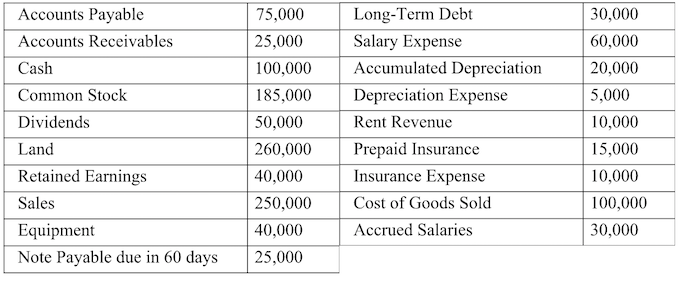# Problem: Listed below are the account balances of the Frank Corporation on December 31, 2014. What are Frank’s total assets and total liabilities on December 31, 2014: a. $420,000;$180,000. b. $420,000;$160,000. c. $440,000;$160,000. d. $460,000;$130,000.

###### Problem Details

Listed below are the account balances of the Frank Corporation on December 31, 2014.

What are Frank’s total assets and total liabilities on December 31, 2014:

a. $420,000;$180,000.

b. $420,000;$160,000.

c. $440,000;$160,000.

d. $460,000;$130,000.